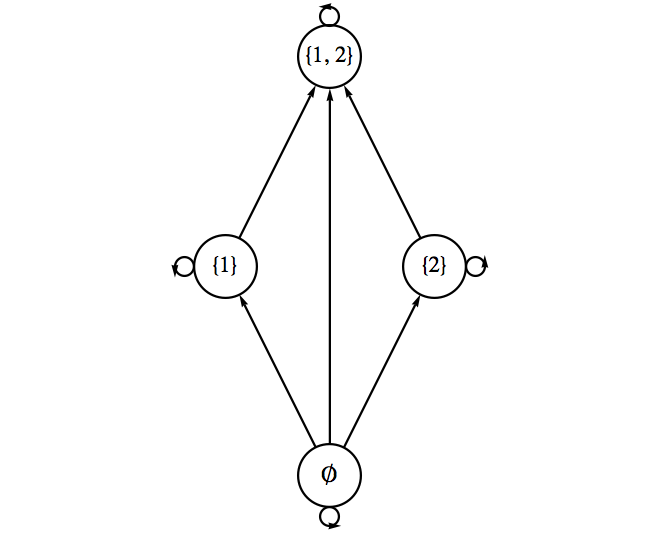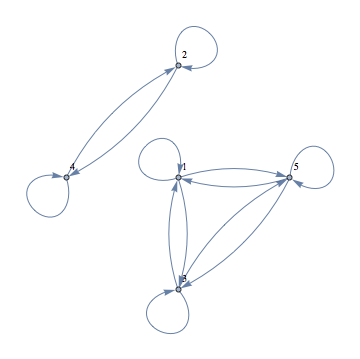# Applied Discrete Structures

## Section6.2Graphs of Relations on a Set

In this section we introduce directed graphs as a way to visualize relations on a set.

### Subsection6.2.1Digraphs

Let $$A = \{0, 1,2,3\}\text{,}$$ and let
\begin{equation*} r = \{(0, 0), (0, 3), (1, 2), (2, 1), (3, 2), (2, 0)\} \end{equation*}
In representing this relation as a graph, elements of $$A$$ are called the vertices of the graph. They are typically represented by labeled points or small circles. We connect vertex $$a$$ to vertex $$b$$ with an arrow, called an edge, going from vertex $$a$$ to vertex $$b$$ if and only if $$a r b\text{.}$$ This type of graph of a relation $$r$$ is called a directed graph or digraph. Figure 6.2.1 is a digraph for $$r\text{.}$$ Notice that since 0 is related to itself, we draw a “self-loop” at 0.
The actual location of the vertices in a digraph is immaterial. The actual location of vertices we choose is called an embedding of a graph. The main idea is to place the vertices in such a way that the graph is easy to read. After drawing a rough-draft graph of a relation, we may decide to relocate the vertices so that the final result will be neater. Figure 6.2.1 could also be presented as in Figure 6.2.2.
A vertex of a graph is also called a node, point, or a junction. An edge of a graph is also referred to as an arc, a line, or a branch. Do not be concerned if two graphs of a given relation look different as long as the connections between vertices are the same in the two graphs.
Consider the relation $$s$$ whose digraph is Figure 6.2.4. What information does this give us? The graph tells us that $$s$$ is a relation on $$A = \{1, 2, 3\}$$ and that $$s = \{(1, 2), (2, 1), (1, 3), (3, 1), (2, 3), (3, 3)\}\text{.}$$Figure 6.2.4. Digraph of the relation $$s$$
We will be building on the next example in the following section.
Let $$B = \{1,2\}\text{,}$$ and let $$A = \mathcal{P}(B) = \{\emptyset, \{1\}, \{2\}, \{1,2\}\}\text{.}$$ Then $$\subseteq$$ is a relation on $$A$$ whose digraph is Figure 6.2.6.Figure 6.2.6. Graph for set containment on subsets of $$\{1,2\}$$
We will see in the next section that since $$\subseteq$$ has certain structural properties that describe “partial orderings.” We will be able to draw a much simpler type graph than this one, but for now the graph above serves our purposes.

### Exercises6.2.2Exercises

#### 1.

Let $$A = \{1, 2, 3, 4\}\text{,}$$ and let $$r$$ be the relation $$\leq$$ on $$A\text{.}$$ Draw a digraph for $$r\text{.}$$

#### 2.

Let $$B = \{1,2, 3, 4, 6, 8, 12, 24\}\text{,}$$ and let $$s$$ be the relation “divides” on $$B\text{.}$$ Draw a digraph for $$s\text{.}$$

#### 3.

Let $$A=\{1,2,3,4,5\}\text{.}$$ Define $$t$$ on $$A$$ by $$a t b$$ if and only if $$b - a$$ is even. Draw a digraph for $$t\text{.}$$Figure 6.2.9. Digraph of the relation $$t$$

#### 4.

Let $$A$$ be the set of strings of 0’s and 1’s of length 3 or less. This includes the empty string, $$\lambda\text{,}$$ which is the only string of length zero.
1. Define the relation of $$d$$ on $$A$$ by $$x d y$$ if $$x$$ is contained within $$y\text{.}$$ For example, $$01 d 101\text{.}$$ Draw a digraph for this relation.
2. Do the same for the relation $$p$$ defined by $$x p y$$ if $$x$$ is a prefix of $$y\text{.}$$ For example, $$10 p 101\text{,}$$ but $$01 p 101$$ is false.

#### 5.

Recall the relation in Exercise 5 of Section 6.1, $$\rho$$ defined on the power set, $$\mathcal{P}(S)\text{,}$$ of a set $$S\text{.}$$ The definition was $$(A,B) \in \rho$$ iff $$A\cap B = \emptyset\text{.}$$ Draw the digraph for $$\rho$$ where $$S = \{a, b\}\text{.}$$

#### 6.

Let $$C= \{1,2, 3, 4, 6, 8, 12, 24\}$$ and define $$t$$ on $$C$$ by $$a t b$$ if and only if $$a$$ and $$b$$ share a common divisor greater than 1. Draw a digraph for $$t\text{.}$$